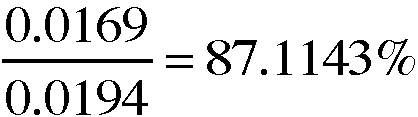# Laboratory Manual for Principles of General Chemistry: Experiment 3 Prelaboratory Assignment: Chemistry of Copper Flashcards

Set Details Share
created 11 years ago by momsaidno
1,816 views
updated 11 years ago by momsaidno
College: First year, College: Second year
Subjects:
chemistry, general chemistry, science
Page to share:
Embed this setcancel
COPY
code changes based on your size selection
Size:
X

1

1. Review net-ionic equations 3.2, 3.4, 3.7, 3.9, and 3.11.
-a. Three of the equations represent oxidation-reduction reactions. Identify the three equations and indicate the oxidizing agent in each.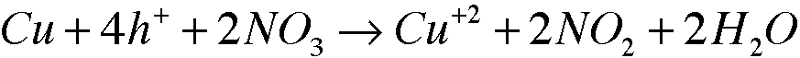1: 3.2

Oxidizing Agent: N

2

1. Review net-ionic equations 3.2, 3.4, 3.7, 3.9, and 3.11.
-a. Three of the equations represent oxidation-reduction reactions. Identify the three equations and indicate the oxidizing agent in each.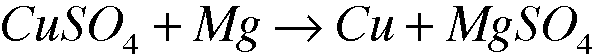2: 3.7

Oxidizing Agent: Mg

3

1. Review net-ionic equations 3.2, 3.4, 3.7, 3.9, and 3.11.
-a. Three of the equations represent oxidation-reduction reactions. Identify the three equations and indicate the oxidizing agent in each.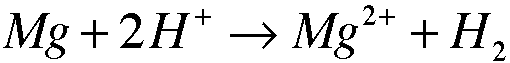3. 3.11

Oxidizing Agent: H

4

2. Copper forms many different compounds in this experiment.
-a. Experimental Procedure, Part A. Identify the oxidizing agent in the conversion of copper metal to copper(II) ion.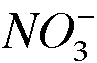The nitrogen in nitric acid (NO_3^-) is the oxidizing agent.

5

2. Copper forms many different compounds in this experiment.
-b. Experimental Procedure, Part E. Identify the oxidizing agent in the conversion of cooper(II) ion to copper metal.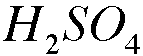The Hydrogen atom in H2SO4 is the oxidizing agent.

6

2. Copper forms many different compounds in this experiment.
-c. Experimental Procedure, Part D. Classify the type of reaction for the conversion of copper(II) oxide to copper(II) sulfate.

It is an oxidation/reduction reaction.

7

3. A number of Caution cautions chemicals and solutions are used in this experiment, Refer to the Experimental Procedure and the corresponding sections to identify the chemical or solution that must handle with care.
Part A.2
Part A.2
Part B.1
Part D.1
Part E.1

Part A.2: HNO3(concentrated)
Part A.2: NO2 gas
Part B.1: NaOH
Part D.1: H2SO4
Part E.1: H2SO4

8

4. Experimental Procedure, part A.2. What volume, in drops, of 16 M (conc) HNO3 is required to react with 0.0214g of Cu metal? See equation 3.1. Assume 20 drops per milliliter.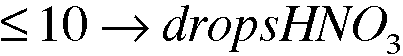9

5. Experimental Procedure, Parts C.1 and E.3. Extreme caution must be observed when hearing a solution in a test tube.
-a. What criterion indicates that you are heating the solution in the test tube with a 'cool flame?'

footnote 2, pg. 76

Not hot enough to be felt by hand when holding tube w/ clamp

10

5. Experimental Procedure, Parts C.1 and E.3. Extreme caution must be observed when hearing a solution in a test tube.
-b. At what angle should the test tube be help while moving the test tube circularly in and out of the cool flame?

45 degrees

11

5. Experimental Procedure, Parts C.1 and E.3. Extreme caution must be observed when hearing a solution in a test tube.
-c. What is the consequence of not using this technique properly?

"Contents maybe ejected violently if not heated correctly."

12

6. a. What function does the centrifuge perform?

It spines and shakes the test tube at an extremely high speed to separate it's contents.

13

6.b. Describe the technique for balancing a centrifuge when centrifuging a sample.

Put each tube at the opposite angle. Like setting plates at a table, try to make sure the able between each is the same.

14

7. A 0.0194-g sample of copper metal is recycled through the series of reactions in this experiment. If 0.0169g of copper is the recovered after the series of reactions in this experiment, what is the percent recovery of the copper metal?